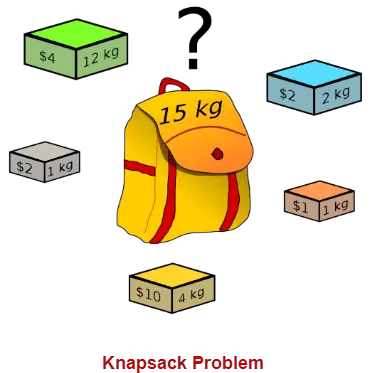# Fractional Knapsack Problem | Greedy Method | Example

## Knapsack Problem-

You are given the following-

• A knapsack (kind of shoulder bag) with limited weight capacity.
• Few items each having some weight and value.

The problem states-

Which items should be placed into the knapsack such that-

• The value or profit obtained by putting the items into the knapsack is maximum.
• And the weight limit of the knapsack does not exceed.## Knapsack Problem Variants-

Knapsack problem has the following two variants-

1. Fractional Knapsack Problem
2. 0/1 Knapsack Problem

## Fractional Knapsack Problem-

In Fractional Knapsack Problem,

• As the name suggests, items are divisible here.
• We can even put the fraction of any item into the knapsack if taking the complete item is not possible.
• It is solved using Greedy Method.

## Fractional Knapsack Problem Using Greedy Method-

Fractional knapsack problem is solved using greedy method in the following steps-

### Step-01:

For each item, compute its value / weight ratio.

### Step-02:

Arrange all the items in decreasing order of their value / weight ratio.

### Step-03:

Start putting the items into the knapsack beginning from the item with the highest ratio.

Put as many items as you can into the knapsack.

## Time Complexity-

• The main time taking step is the sorting of all items in decreasing order of their value / weight ratio.
• If the items are already arranged in the required order, then while loop takes O(n) time.
• The average time complexity of Quick Sort is O(nlogn).
• Therefore, total time taken including the sort is O(nlogn).

## Problem-

For the given set of items and knapsack capacity = 60 kg, find the optimal solution for the fractional knapsack problem making use of greedy approach.

 Item Weight Value 1 5 30 2 10 40 3 15 45 4 22 77 5 25 90

OR

Find the optimal solution for the fractional knapsack problem making use of greedy approach. Consider-

n = 5

w = 60 kg

(w1, w2, w3, w4, w5) = (5, 10, 15, 22, 25)

(b1, b2, b3, b4, b5) = (30, 40, 45, 77, 90)

OR

A thief enters a house for robbing it. He can carry a maximal weight of 60 kg into his bag. There are 5 items in the house with the following weights and values. What items should thief take if he can even take the fraction of any item with him?

 Item Weight Value 1 5 30 2 10 40 3 15 45 4 22 77 5 25 90

## Solution-

### Step-01:

Compute the value / weight ratio for each item-

 Items Weight Value Ratio 1 5 30 6 2 10 40 4 3 15 45 3 4 22 77 3.5 5 25 90 3.6

### Step-02:

Sort all the items in decreasing order of their value / weight ratio-

I1          I2          I5          I4          I3

(6)       (4)        (3.6)      (3.5)       (3)

### Step-03:

Start filling the knapsack by putting the items into it one by one.

 Knapsack Weight Items in Knapsack Cost 60 Ø 0 55 I1 30 45 I1, I2 70 20 I1, I2, I5 160

Now,

• Knapsack weight left to be filled is 20 kg but item-4 has a weight of 22 kg.
• Since in fractional knapsack problem, even the fraction of any item can be taken.
• So, knapsack will contain the following items-

< I1 , I2 , I5 , (20/22) I4 >

Total cost of the knapsack

= 160 + (20/27) x 77

= 160 + 70

= 230 units

## Important Note-

Had the problem been a 0/1 knapsack problem, knapsack would contain the following items-

< I1 , I2 , I5 >

The knapsack’s total cost would be 160 units.

To gain better understanding about Fractional Knapsack Problem,

Watch this Video Lecture

Next Article- Job Sequencing With Deadlines

Get more notes and other study material of Design and Analysis of Algorithms.

Watch video lectures by visiting our YouTube channel LearnVidFun.

SummaryArticle Name
Fractional Knapsack Problem | Greedy Method | Example
Description
Fractional Knapsack Problem is a variant of Knapsack Problem that allows to fill the knapsack with fractional items. Fractional Knapsack Problem solved using Greedy Method. Fractional Knapsack Problem Example & Algorithm.
Author
Publisher Name
Gate Vidyalay
Publisher Logo